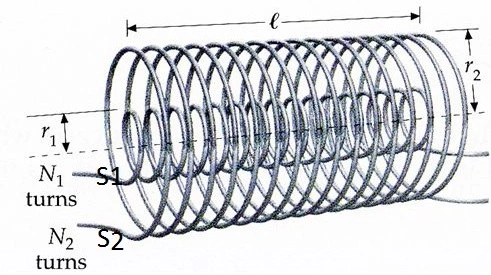# Category Physics class -12 derivations

## Derivation for mutual inductance of two long co-axial solenoidsIn this article, we will derive an expression for the mutual inductance of two long coaxial solenoids. So let’s get started… What is mutual inductance? Mutual inductance is a phenomenon in which an emf is induced in the secondary coil…

## Use Ampere’s circuital law to derive an expression for the magnetic field due to a long straight wireIn this article, we will derive an expression for the magnetic field due to the long straight current-carrying conductor using Ampere’s circuital law. So without talking more, let’s start… AMPERE’S CIRCUITAL LAW: A SNAPSHOT Let’s recall what Ampere’s circuital law…

## Derive an expression for magnetic field on the axis of a circular current carrying loop, Class 12In this article we are going to derive an expression for magnetic field on the axis of circular current carrying loop. So without talking so much let’s gets started… MAGNETIC FIELD ON THE AXIS OF A CIRCULAR CURRENT CARRYING LOOP…